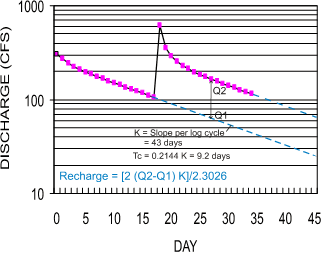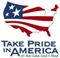USGS Groundwater Information

Groundwater Resources Program

# Recession-Curve Displacement Method for Estimating Groundwater Recharge In Humid Regions

The recession-curve displacement method can be used to estimate groundwater recharge from streamflow records. The method is based on the premise that the streamflow-recession curve is displaced upward during periods of groundwater recharge. Ground-water recharge during an event can be estimated on the basis of work by Rorabaugh (1964) and Glover (1964) , both of whom derived analytical equations for instantaneous, one-dimensional groundwater discharge to a fully penetrating stream in an idealized, homogenous aquifer. These equations showed that the total potential groundwater discharge to the stream at a specific critical time after the peak streamflow is equal to about one-half the volume of groundwater recharged during the peak. Thus, recharge from a specific event can be derived (Rutledge, 1998, p. 19) as:

 R = 2 (Q2-Q1) K (1) 2.3026
Where:

R is total volume of recharge for the streamflow peak (cubic feet);
Q1 is groundwater discharge at critical time (T c ) extrapolated from pre-storm recession (cubic feet per day);
Q2 is groundwater discharge at critical time (T c ) extrapolated from post-storm recession (cubic feet per day); and
K is the recession index, defined as the time required for groundwater discharge to decline by one log cycle after the recession curve becomes nearly linear on a semilog hydrograph (days).Example application of the recession-curve displacement method.

An example of the recession-curve displacement method is illustrated, where Q1 is 65 cubic feet per second (cfs), Q2 is 165cfs, K is 43 days, and critical time is 9.2 days. From equation 1, groundwater recharge for that single event is 323 million cubic feet. Recharge from that event could be expressed in inches by dividing by the watershed area upstream of the streamflow-gaging station and converting units.

Manual computation of recharge with the recession-curve displacement method was cumbersome and not widely used, so the procedure was automated by Rutledge (1998) in the computer program RORA. Application of the recession-curve displacement method with RORA requires two basic steps:

Although the recession-curve displacement method uses streamflow data to estimate groundwater recharge, it is not a "base-flow separation" technique. The method has a theoretical foundation based on a simple hydrologic model described above, which contrasts markedly to the more subjective base-flow separation methods. Because of the simple, theoretical foundation, there are several key assumptions and critical issues that should be evaluated to determine the applicability of the method for a particular problem.U.S. Department of the Interior | U.S. Geological Survey
URL: http://water.usgs.gov/ogw/gwrp/methods/recession_curve/index.html
Page Contact Information: Contact the Groundwater Resources Program